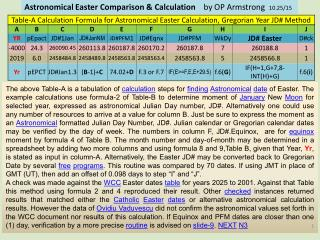DownloadDownload PresentationAstronomical & Gregorian Easter date using Julian Day Numbers

# Astronomical & Gregorian Easter date using Julian Day Numbers

Télécharger la présentation## Astronomical & Gregorian Easter date using Julian Day Numbers

- - - - - - - - - - - - - - - - - - - - - - - - - - - E N D - - - - - - - - - - - - - - - - - - - - - - - - - - -
##### Presentation Transcript

1. Astronomical Easter Comparison & Calculation by OP Armstrong 10.25/15 Table-A Calculation Formula for Astronomical Easter Calculation, Gregorian Year JD# Method A B C D E F YR pEpact JD#1Jan JD#JanNM JD#PFM1 JD#Eqnx -4000 24.3 260090.45 260113.8 260187.8 260170.2 2019 6.0 2458484.8 2458489.8 2458563.8 2458563.4 G H I J JD# Easter 260188.8 2458566.8 IF(H=1,G+7,8- INT(H)+G) JD#PFM 260187.8 2458563.8 WkDy 7 5 D#ck 1 1 74.02+D F.3 or F.7 IF(E>=F,E,E+29.5) f.6(G) Yr pEPCT JD#Jan1.3 (B-1)+C f.6(i) The above Table-A is a tabulation of calculation steps for finding Astronomical date of Easter. The example calculations use formula-2 of Table-B to determine moment of January New Moon for selected year, expressed as astronomical Julian Day number, JD#. Alternatively one could use any number of resources to arrive at a value for column B. Just be sure to express the moment as an Astronomical formatted Julian Day Number, JD#. Julian calendar or Gregorian calendar dates may be verified by the day of week. The numbers in column F, JD#.Equinox, are for equinox moment by formula 4 of Table B. The month number and day-of-month may be determined in a spreadsheet by adding two more columns and using formula 8 and 9,Table B, given that Year, Yr, is stated as input in column-A. Alternatively, the Easter JD# may be converted back to Gregorian Date by several free programs. This routine was compared by 70 dates. If using JMT in place of GMT (UT), then add an offset of 0.098 days to step “I” and “J”. A check was made against the WCC Easter dates table for years 2025 to 2001. Against that Table this method using formula 2 and 4 reproduced their result. Other checked instances returned results that matched either the Catholic Easter dates or alternative astronomical calculation results. However the data of Ovidiu Vaduvescu did not confirm the astronomical values set forth in the WCC document nor results of this calculation. If Equinox and PFM dates are closer than one (1) day, verification by a more precise routine is advised on slide-9. NEXT N3 1

2. Astronomical Easter Comparison & Calculation by OP Armstrong 9.5/15 Name (Nu) TABLE-B Excel Astronomical Name Formula: Yr-year; JD#-Julian Day 29.09-MOD(MOD(Yr,19)*11-INT((Yr-1502.57-12*MOD(Yr,19))/228),29.983) pEpact.Cassidy.f1 29.5-MOD(MOD(Yr,19)*11-INT((Yr-1584-12*MOD(Yr,19))/228),30) pEpact.Cassidy.0.f1b IF((1+MOD((365.242454*(-4006-Yr)),29.5306))>=30,((1+MOD((365.242454*(-4006- Yr)),29.5306))-30),(1+MOD((365.242454*(-4006-Yr)),29.5306))) pEpact.Lunation#.f2 JD# Jan1.f3 257898.52-365.242454*(-4006-Yr) (2457102.448+(Yr-2015)*365.2422)+((-0.0005947871)*((Yr-2015)/1000)^4+(- 0.00392591)*((Yr-2015)/1000)^3+(0.013808751)*((Yr- 2015)/1000)^2+(0.1590901)*((Yr-2015)/1000)) JD# Equinox.f4 1stPage March 1stMoon.f5 JD#.Jan1 + pEpact + 59 Day of Week.f6 (1+INT(MOD((1.5+JD#),7))) one is Sunday and 7 is Saturday, etc 257978.00-365.242454*(-4006-Yr) JD#21March.f7 1+INT(MOD(((INT(MOD(((INT(JD#+0.5)+(- 37+INT(0.5+0.75*INT((INT(JD#+0.5)-4479.5)/36524.25))))- 59.25),365.25)))+0.5),30.6)) Day of Month.f8 1+MOD((2+INT(((INT(MOD(((INT(JD#+0.5)+(- 37+INT(0.5+0.75*INT((INT(JD#+0.5)-4479.5)/36524.25))))- 59.25),365.25)))+0.5)/30.6)),12) Month Number.f9 , 3=March, 4=April 2 Next page